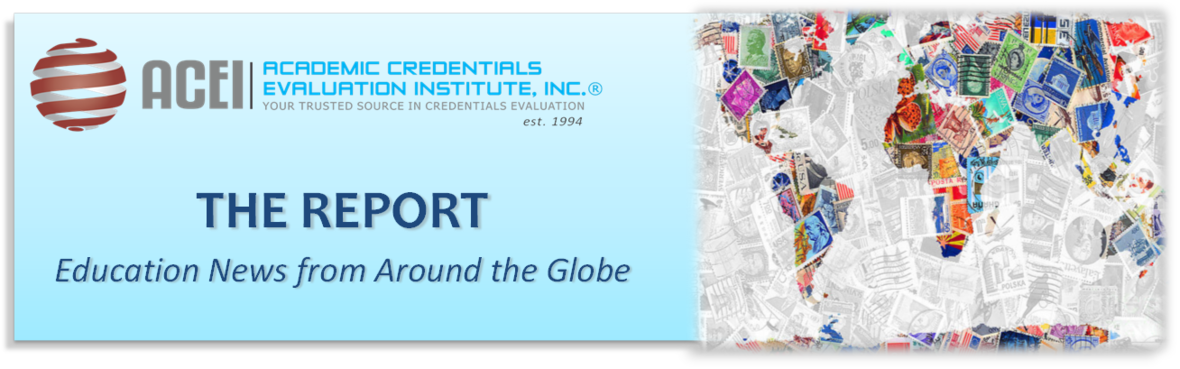# December 2018 - The Report

As we approach the end of 2018, we wish to thank you for your subscription and support of ACEI’s monthly Newsletter The Report. Our team works hard to bring to you timely and relevant topics on education, technology, politics, culture and trends from around the globe. We shall strive to bring you more of the same in 2019.

From all of us here at ACEI, we wish you and yours a Happy Holiday Season and a New Year filled with health, happiness, and spectacular success. Cheers!

 table div table+table+table div table{width:100%;padding:0}table div table+table+table div table img{width:96.23%;padding:0;float:none}table div table+table+table div table td{width:100%;padding:0 1.88% 18px}/* styles */# ACEI News

## PARTNERSHIP PROGRAMS

The Welcome Project© is a joint endeavor between ACEI and iTEP International offering individuals classified as refugees assistance with the evaluation of their academic credentials for U.S. educational equivalence and determine their English language proficiency through an approved language assessment test.

The Welcome Project combines both the international credential evaluation and English language proficiency testing to assist individuals at the onset of their resettlement in the U.S. These individuals will be able to receive recognition of their educational achievements and language competency. Such recognition will enable them to integrate into their new adopted community much faster as they set out to pursue employment opportunities and further their education. For more information on how you can participate in The Welcome Project, please click here.

Scottish Qualifications Authority
ACEI is proud to announce its partnership with the Scottish Qualifications Authority (SQA) through the publication of the ACEI’s Advisory Services ‘Program/Curriculum Review’ Report, which provides detailed analysis of four of SQA’s Advanced Qualifications. The report, based on ACEI’s key principles in international credential/program evaluation, gives higher education institutions in the United States a better understanding of the value of SQA qualifications, the caliber of those who have achieved them, and how they compare with programs of study offered in universities and colleges across the United States. For more information on the SQA, please contact ACEI’s President & CEO Jasmin Saidi-Kuehnert at jasmin@acei-global.org.

## PARTNERSHIP OPPORTUNITIES

Interested in partnership opportunities with ACEI? Please email Yolinisse Moreno, Director of Communications at acei@acei-global.org or 310-275-3530.

 table div table+table+table+table+table+table+table div table{width:100%;padding:0}table div table+table+table+table+table+table+table div table img{width:96.23%;padding:0;float:none}table div table+table+table+table+table+table+table div table td{width:100%;padding:0 1.88% 18px}/* styles */table div table+table+table+table+table+table+table+table+table div table{width:100%;padding:0}table div table+table+table+table+table+table+table+table+table div table table{padding:0;float:left!important;width:37.924%!important}table div table+table+table+table+table+table+table+table+table div table table+table td,table div table+table+table+table+table+table+table+table+table div table td{padding-left:0;padding-right:0}table div table+table+table+table+table+table+table+table+table div table table td{padding-left:0;padding-right:20px}table div table+table+table+table+table+table+table+table+table div table table+table{float:left!important;width:62.076%!important}/* styles */
 table.module-9{width:77.17%;padding:0}table div table+table+table+table+table+table+table+table+table+table div table{width:77.17%;float:none;margin-left:auto;margin-right:auto;padding:0}table div table+table+table+table+table+table+table+table+table+table div table a{border:0 none;text-decoration:none}table div table+table+table+table+table+table+table+table+table+table div table img{width:100%!important;border:0 none;text-decoration:none}table div table+table+table+table+table+table+table+table+table+table div table td{width:100%;padding:0}/* styles */
 table div table+table+table+table+table+table+table+table+table+table+table div table{width:100%;padding:0}table div table+table+table+table+table+table+table+table+table+table+table div table img{width:96.23%;padding:0;float:none}table div table+table+table+table+table+table+table+table+table+table+table div table td{width:100%;padding:0 1.88% 18px}/* styles */# News Around the worldBrazil’s president-elect, Jair Bolsonaro. Photograph: Marcelo Sayao/EPA

# Australian University to Help Indian Farmers Double their Income by 2022

An Australian university is planning to invest AUD 5 million as part of a collaboration with agricultural universities across India, to leverage research and innovations that may help Indian farmers double their income by 2022. To read more, click here

 table div table+table+table+table+table+table+table+table+table+table+table+table+table+table+table div table{width:100%;padding:0}table div table+table+table+table+table+table+table+table+table+table+table+table+table+table+table div table img{width:96.23%;padding:0;float:none}table div table+table+table+table+table+table+table+table+table+table+table+table+table+table+table div table td{width:100%;padding:0 1.88% 18px}/* styles */Photo: Colombia Informa

# The Tale of 3 Countries and Fake Degrees

In a recent post on ACEI-Global.BLOG we shared news of the arrests of 40 individuals in Israel for having fake degrees from three accredited universities in Armenia and a UGC-approved University in India also accused of fraud. To read more, click here

 table div table+table+table+table+table+table+table+table+table+table+table+table+table+table+table+table+table+table div table{width:100%;padding:0}table div table+table+table+table+table+table+table+table+table+table+table+table+table+table+table+table+table+table div table img{width:96.23%;padding:0;float:none}table div table+table+table+table+table+table+table+table+table+table+table+table+table+table+table+table+table+table div table td{width:100%;padding:0 1.88% 18px}/* styles */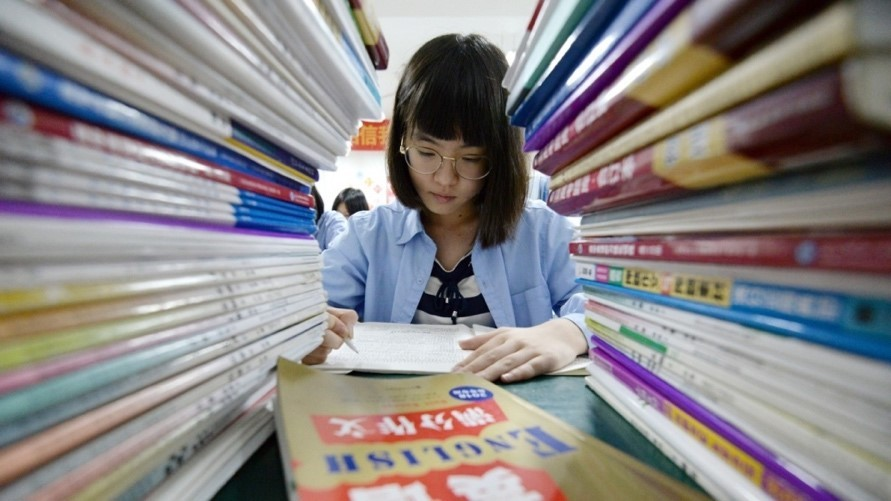# Chinese parents spend \$43,000 on after school classes

A recent report shows that Chinese parents spend \$43,000 on after school classes for their children. To read more, click here

 table div table+table+table+table+table+table+table+table+table+table+table+table+table+table+table+table+table+table+table+table+table div table{width:100%;padding:0}table div table+table+table+table+table+table+table+table+table+table+table+table+table+table+table+table+table+table+table+table+table div table img{width:96.23%;padding:0;float:none}table div table+table+table+table+table+table+table+table+table+table+table+table+table+table+table+table+table+table+table+table+table div table td{width:100%;padding:0 1.88% 18px}/* styles */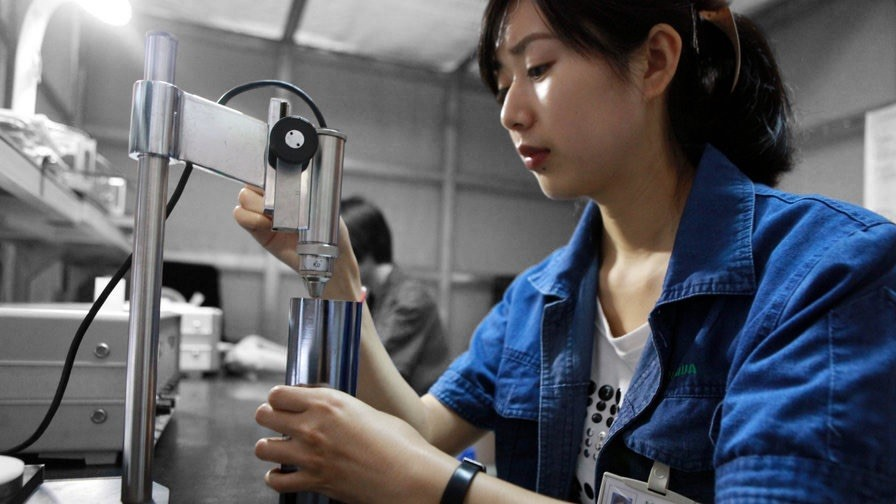# Taking the lead in Scientific Research

According to the journal Learned Publishing, American scientists published 409,000 science, technology and medical papers in 2017, while China published 426,000. To read more, click here

 table div table+table+table+table+table+table+table+table+table+table+table+table+table+table+table+table+table+table+table+table+table+table+table+table div table{width:100%;padding:0}table div table+table+table+table+table+table+table+table+table+table+table+table+table+table+table+table+table+table+table+table+table+table+table+table div table img{width:96.23%;padding:0;float:none}table div table+table+table+table+table+table+table+table+table+table+table+table+table+table+table+table+table+table+table+table+table+table+table+table div table td{width:100%;padding:0 1.88% 18px}/* styles */Teachers protesting in Marseilles/AP

# Building the World’s Largest Digital Library

Egypt, Saudi Arabia and the UAE have joined forces to form the world’s largest digital library.

 table div table+table+table+table+table+table+table+table+table+table+table+table+table+table+table+table+table+table+table+table+table+table+table+table+table+table div table{width:100%;padding:0}table div table+table+table+table+table+table+table+table+table+table+table+table+table+table+table+table+table+table+table+table+table+table+table+table+table+table div table img{width:96.23%;padding:0;float:none}table div table+table+table+table+table+table+table+table+table+table+table+table+table+table+table+table+table+table+table+table+table+table+table+table+table+table div table td{width:100%;padding:0 1.88% 18px}/* styles */# Colleges of Education Reopen after Strikes End

All 46 Colleges of Education in the country are expected to resume today following a directive by the Ministry of Education. To read more, click here

 table div table+table+table+table+table+table+table+table+table+table+table+table+table+table+table+table+table+table+table+table+table+table+table+table+table+table+table+table div table{width:100%;padding:0}table div table+table+table+table+table+table+table+table+table+table+table+table+table+table+table+table+table+table+table+table+table+table+table+table+table+table+table+table div table img{width:96.23%;padding:0;float:none}table div table+table+table+table+table+table+table+table+table+table+table+table+table+table+table+table+table+table+table+table+table+table+table+table+table+table+table+table div table td{width:100%;padding:0 1.88% 18px}/* styles */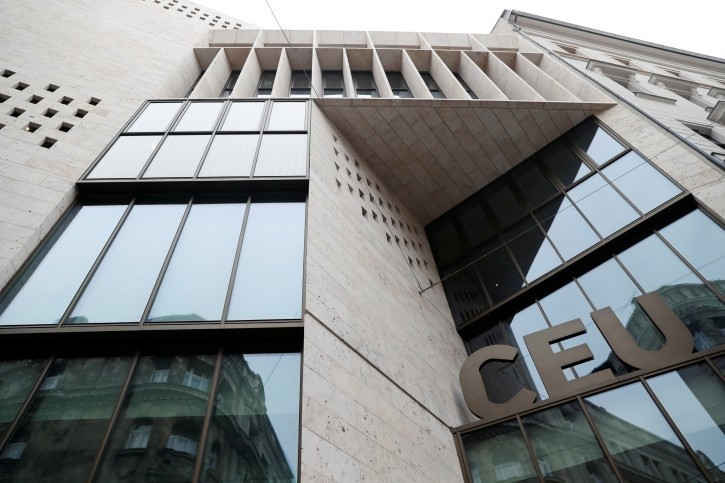# Central European University Forced Out of Hungary

The Central European University (CEU) has announced that it has been forced to launch all new United States-accredited degree programs in Vienna, Austria, in September 2019 instead of Budapest, Hungary. To read more, click here

And, be sure to read a blog we wrote on CEU that offers background information on the University. Click here

 table div table+table+table+table+table+table+table+table+table+table+table+table+table+table+table+table+table+table+table+table+table+table+table+table+table+table+table+table+table+table+table div table{width:100%;padding:0}table div table+table+table+table+table+table+table+table+table+table+table+table+table+table+table+table+table+table+table+table+table+table+table+table+table+table+table+table+table+table+table div table img{width:96.23%;padding:0;float:none}table div table+table+table+table+table+table+table+table+table+table+table+table+table+table+table+table+table+table+table+table+table+table+table+table+table+table+table+table+table+table+table div table td{width:100%;padding:0 1.88% 18px}/* styles */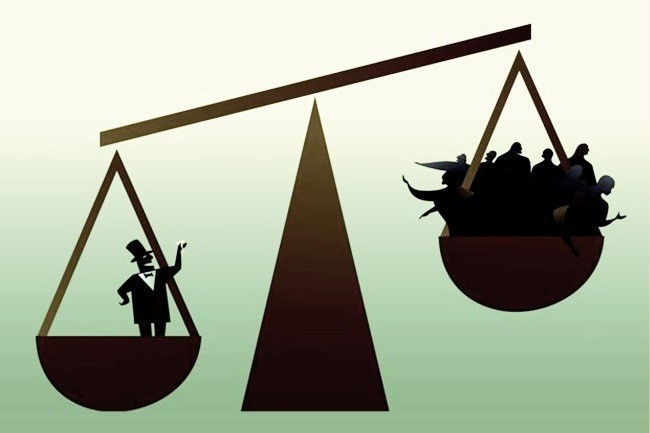(Nelvin C. Cepeda / San Diego Union-Tribune)

## IRELAND

A recent survey reveals that most schools in better-off parts of Dublin had progression rates of 90% or more. By contrast, individual schools in more deprived parts of the capital had progression rates as low as 7%. These figures indicate both the extent of middle-class privilege and the inequality that continues to pervade the education system despite decades of investment in deprived schools. To read more, click here

An article from May 2015 written by Prof. Simon Marginson, at the University of London, UK, asks if hierarchical higher education is fueled by the wealth gap? Prof. Marginson looks at the U.S. with it’s extreme levels of economic and social inequality and reports that higher education progress on access seems to have halted. Seeing the similar trend happening in Ireland, this article offers additional insight and perspective. To read more, click here

 table div table+table+table+table+table+table+table+table+table+table+table+table+table+table+table+table+table+table+table+table+table+table+table+table+table+table+table+table+table+table+table+table+table+table div table{width:100%;padding:0}table div table+table+table+table+table+table+table+table+table+table+table+table+table+table+table+table+table+table+table+table+table+table+table+table+table+table+table+table+table+table+table+table+table+table div table img{width:96.23%;padding:0;float:none}table div table+table+table+table+table+table+table+table+table+table+table+table+table+table+table+table+table+table+table+table+table+table+table+table+table+table+table+table+table+table+table+table+table+table div table td{width:100%;padding:0 1.88% 18px}/* styles */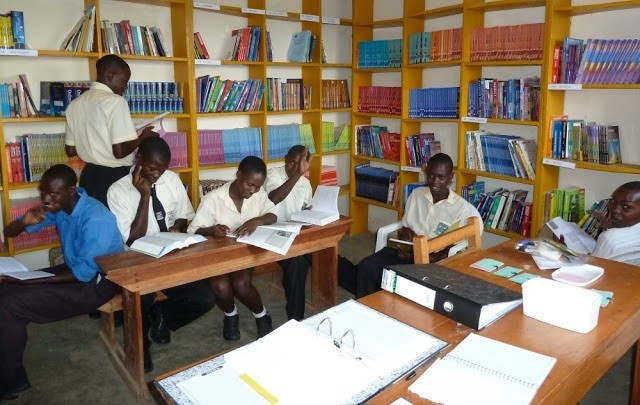# Literacy Troubles

 table div table+table+table+table+table+table+table+table+table+table+table+table+table+table+table+table+table+table+table+table+table+table+table+table+table+table+table+table+table+table+table+table+table+table+table+table div table{width:100%;padding:0}table div table+table+table+table+table+table+table+table+table+table+table+table+table+table+table+table+table+table+table+table+table+table+table+table+table+table+table+table+table+table+table+table+table+table+table+table div table img{width:96.23%;padding:0;float:none}table div table+table+table+table+table+table+table+table+table+table+table+table+table+table+table+table+table+table+table+table+table+table+table+table+table+table+table+table+table+table+table+table+table+table+table+table div table td{width:100%;padding:0 1.88% 18px}/* styles */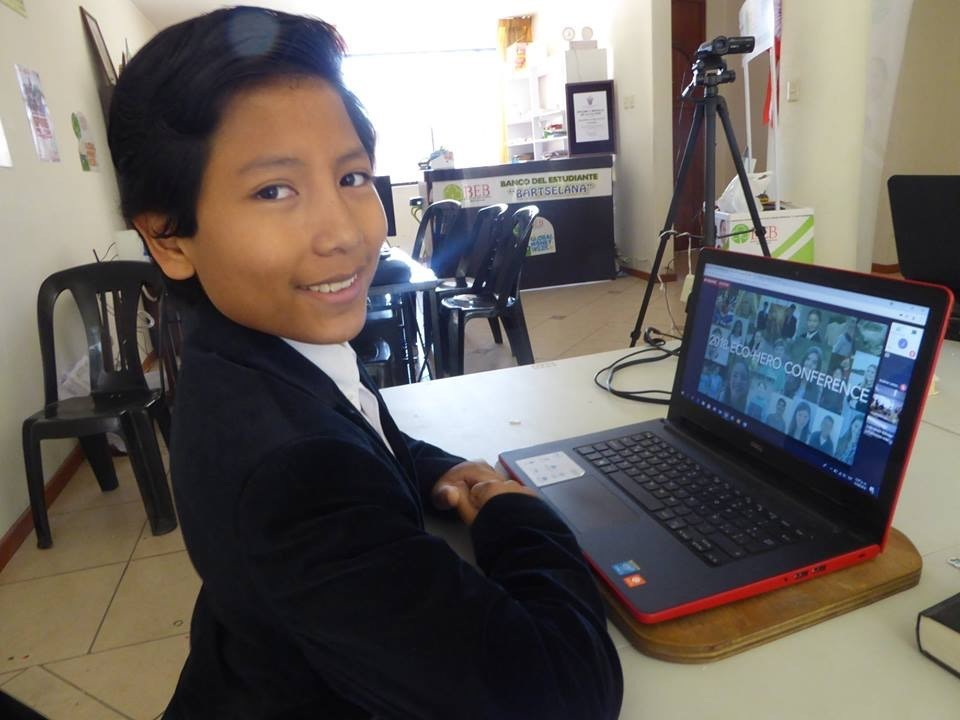# An Eco-Bank Created by a Child for Children

Established in 2012, The Bartselana Student Bank is the world’s first cooperative bank for kids. It’s founder, Jose Adolfo Quisocola, came up with the innovative idea of an eco-bank when he was just seven. The eco-bank allows kids of all ages to become economically independent and financially savvy – while also helping the environment. To read more, click here

 table div table+table+table+table+table+table+table+table+table+table+table+table+table+table+table+table+table+table+table+table+table+table+table+table+table+table+table+table+table+table+table+table+table+table+table+table+table+table div table{width:100%;padding:0}table div table+table+table+table+table+table+table+table+table+table+table+table+table+table+table+table+table+table+table+table+table+table+table+table+table+table+table+table+table+table+table+table+table+table+table+table+table+table div table img{width:96.23%;padding:0;float:none}table div table+table+table+table+table+table+table+table+table+table+table+table+table+table+table+table+table+table+table+table+table+table+table+table+table+table+table+table+table+table+table+table+table+table+table+table+table+table div table td{width:100%;padding:0 1.88% 18px}/* styles */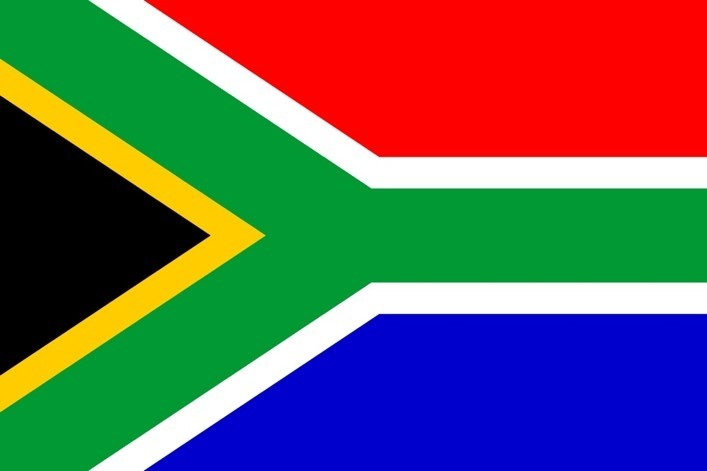# Concern over Fake Qualifications

Misrepresentation of qualifications is a major concern in the public and private sectors, which has prompted the passage of a bill strengthening the ability of South African Qualifications Authority (SAQA ) to verify qualifications. To read more, click here

 table div table+table+table+table+table+table+table+table+table+table+table+table+table+table+table+table+table+table+table+table+table+table+table+table+table+table+table+table+table+table+table+table+table+table+table+table+table+table+table+table div table{width:100%;padding:0}table div table+table+table+table+table+table+table+table+table+table+table+table+table+table+table+table+table+table+table+table+table+table+table+table+table+table+table+table+table+table+table+table+table+table+table+table+table+table+table+table div table img{width:96.23%;padding:0;float:none}table div table+table+table+table+table+table+table+table+table+table+table+table+table+table+table+table+table+table+table+table+table+table+table+table+table+table+table+table+table+table+table+table+table+table+table+table+table+table+table+table div table td{width:100%;padding:0 1.88% 18px}/* styles */## UNITED KINGDOM

According to official figures released earlier this year, British universities have been awarding higher-class degrees at an unprecedented rate over the past decade, with at least one university issuing five times as many first-class degrees last year as it did a decade before. To read more, click here

 table div table+table+table+table+table+table+table+table+table+table+table+table+table+table+table+table+table+table+table+table+table+table+table+table+table+table+table+table+table+table+table+table+table+table+table+table+table+table+table+table+table+table+table div table{width:100%;padding:0}table div table+table+table+table+table+table+table+table+table+table+table+table+table+table+table+table+table+table+table+table+table+table+table+table+table+table+table+table+table+table+table+table+table+table+table+table+table+table+table+table+table+table+table div table img{width:96.23%;padding:0;float:none}table div table+table+table+table+table+table+table+table+table+table+table+table+table+table+table+table+table+table+table+table+table+table+table+table+table+table+table+table+table+table+table+table+table+table+table+table+table+table+table+table+table+table+table div table td{width:100%;padding:0 1.88% 18px}/* styles */# Upcoming Events in December 2018/* styles */ Holiday Schedule ACEI Offices will be closed: December 31st 2018 & January 1, 2019
 table div table+table+table+table+table+table+table+table+table+table+table+table+table+table+table+table+table+table+table+table+table+table+table+table+table+table+table+table+table+table+table+table+table+table+table+table+table+table+table+table+table+table+table+table+table+table+table div table{width:100%;padding:0}table div table+table+table+table+table+table+table+table+table+table+table+table+table+table+table+table+table+table+table+table+table+table+table+table+table+table+table+table+table+table+table+table+table+table+table+table+table+table+table+table+table+table+table+table+table+table+table div table img{width:96.23%;padding:0;float:none}table div table+table+table+table+table+table+table+table+table+table+table+table+table+table+table+table+table+table+table+table+table+table+table+table+table+table+table+table+table+table+table+table+table+table+table+table+table+table+table+table+table+table+table+table+table+table+table div table td{width:100%;padding:0 1.88% 18px}/* styles */table.module-48{width:84.72%;padding:0}table div table+table+table+table+table+table+table+table+table+table+table+table+table+table+table+table+table+table+table+table+table+table+table+table+table+table+table+table+table+table+table+table+table+table+table+table+table+table+table+table+table+table+table+table+table+table+table+table+table div table{width:84.72%;float:none;margin-left:auto;margin-right:auto;padding:0}table div table+table+table+table+table+table+table+table+table+table+table+table+table+table+table+table+table+table+table+table+table+table+table+table+table+table+table+table+table+table+table+table+table+table+table+table+table+table+table+table+table+table+table+table+table+table+table+table+table div table a{border:0 none;text-decoration:none}table div table+table+table+table+table+table+table+table+table+table+table+table+table+table+table+table+table+table+table+table+table+table+table+table+table+table+table+table+table+table+table+table+table+table+table+table+table+table+table+table+table+table+table+table+table+table+table+table+table div table img{width:100%!important;border:0 none;text-decoration:none}table div table+table+table+table+table+table+table+table+table+table+table+table+table+table+table+table+table+table+table+table+table+table+table+table+table+table+table+table+table+table+table+table+table+table+table+table+table+table+table+table+table+table+table+table+table+table+table+table+table div table td{width:100%;padding:0}/* styles */

# Digital Delivery of Evaluation Reports: SecurePathway©:

ACEI is a proud signatory of the Groningen Declaration Network which “seeks common ground in best serving the academic and professional mobility needs of citizens worldwide by bringing together key stakeholders in the Digital Student Data Ecosystem.” For the past two years, ACEI has committed itself to this shared mission through the introduction of SecurePathway©, an ACEI proprietary digital platform for the secure delivery of official evaluation reports and supporting educational credentials. For information on ACEI’s SecurePathway©.

Signing up with ACEI’s SecurePathway©, is free and does not cost the institutions any fees. To receive ACEI expert reports and certified academic credentials via SecurePathway©, complete this form.

*SecurePathway Registration Form*table div table+table+table+table+table+table+table+table+table+table+table+table+table+table+table+table+table+table+table+table+table+table+table+table+table+table+table+table+table+table+table+table+table+table+table+table+table+table+table+table+table+table+table+table+table+table+table+table+table+table+table+table+table+table+table div table{width:100%;padding:0}table div table+table+table+table+table+table+table+table+table+table+table+table+table+table+table+table+table+table+table+table+table+table+table+table+table+table+table+table+table+table+table+table+table+table+table+table+table+table+table+table+table+table+table+table+table+table+table+table+table+table+table+table+table+table+table div table img{width:96.23%;padding:0;float:none}table div table+table+table+table+table+table+table+table+table+table+table+table+table+table+table+table+table+table+table+table+table+table+table+table+table+table+table+table+table+table+table+table+table+table+table+table+table+table+table+table+table+table+table+table+table+table+table+table+table+table+table+table+table+table+table div table td{width:100%;padding:0 1.88% 18px}/* styles */